## KS2 maths 2011 paper B question 4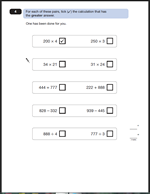We continue our look at the KS2 Maths 2011 Paper B with this question, which should be an easy 2 marks, but all too often little errors are made when using a calculator.

Two marks awarded for all four rows correct.

One mark awarded for 3 out of 4.

No marks for 2 or less correct.

Other clear indications of the answer, such as a cross or Y will also gain the mark.

Suggested method:

The key to answering this question is to remember that a calculator is available and to take each pair of questions separately.

Children should be able to use the keys properly. They should know how to clear the calculator, how to enter numbers and operations and how to read the results. Interpreting the results is also important and it is essential to look at the answer to check that it is sensible – within a range of what is expected.

For example 34 x 21 is going to be around 600 or 700.

With these questions it is a good idea to jot down each of the two answers and then compare them. It is essential that the calculator is cleared after each calculation.

Question 4 from SAT Paper B 2011

Question 4 answers and suggested method 2011 paper B

## Year 5 addition: missing digits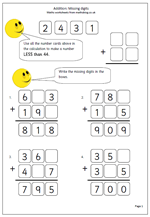On the face of it this might look like an easy page, but in fact many children find this quite tricky. It should not be tried until children have a good grasp of the standard written method of addition.The addition calculation has been laid out in the standard way, together with the answer. However, two of the digits in the sum are missing. Sometimes it is easy to find the missing digit, but not always, especially when tens have been ‘carried’ into hundreds.

If children get this page correct it shows a good understanding of the standard method of addition. If they struggle it would be well worth looking again at this method.

## Year 3 mental arithmetic: addition and subtraction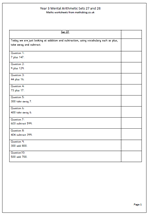This week’s sets of mental arithmetic questions concentrate solely on addition and subtraction. There are many different strategies that can be used, depending very much on the numbers involved.

For example:

7 plus 147 can be done quickly by counting on 7, or by  knowing off by heart that 7 and 7 is 14

9 plus 129 can be done by adding 10 and subtracting 1

44 plus 16 can be done by adding the tens first and then the units, or vice versa.

It is important that children have all these techniques at their fingertips, and one way to make sure they have is to point out the many possible ways and which are the most efficient.

Year 3 mental arithmetic (sets 27 and 28)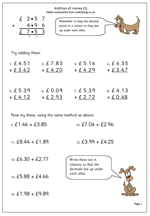I am often asked to provide more pages of addition ‘sums’ so here is another look at using the standard method of addition for money.

The first eight questions are laid out in the correct way. The next seven need to be written in the same way, with the decimal points lining up in a vertical line.

With these questions there is ‘carrying’ from both the hundredths to the tenths and from the tenths to the whole pounds.

If this page is sent as homework look for clues that the answers have been done on a calculator. All working should be shown, including the smaller ‘1’ below the answer line. Also question 10 should be answered as £10.00 rather than just £10.

There is no harm in using a calculator to check that the answers are correct, but the main purpose of pages like these is to give practice and reinforcement of the written method.

## KS2 Maths 2011 Paper B: Question 3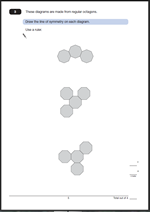Symmetry is a very popular subject with the SAT writers and can be an easy way to pick up a couple of marks.

For this question two marks are awarded for all three lines of symmetry drawn correctly.
One mark awarded for two diagrams completed correctly.
No marks for just one marked correctly.
Slight inaccuracies in drawing can be allowed, provided the intention is clear. A ruler should be used.
Suggested method:
This is very much a case of using the resources that you have been provided with and recognising how they can be useful.

A mirror is certainly helpful for this question. Placing it where it is thought the line of symmetry should be and looking to see if the reflection matches the drawing. When working out where the lines should go imagine that they are folded along the line and completely overlap each other. Some children will find this very tricky, especially as two of the lines of symmetry are not horizontal or vertical.
Alternatively, use tracing paper to draw the diagrams and then fold so that the two sides completely overlap. Draw the line where the fold is. Tracing paper is often provided in the test.

Question 3

Question 3 answer and suggested method 2011 paper B

## Resource of the week: Maths Vocabulary for Year 1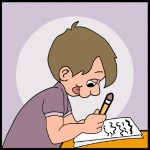I am often asked about the words and ideas that children should be familiar with by the end of Year 1 and there is an excellent set of pages which help with this, found in the Year 1 Maths Worksheets/Vocabulary section.

If a child does not respond to a mathematical question it may be because they do not understand the words or instructions used. Another difficulty can arise when a mathematical term has a different meaning in everyday English eg ‘table’.

The following list has the new words that they should come across in school during year 1 to do with calculating and solving problems.
Words to do with calculating and solving problems:
plus                                       near double
how much more is…?     how much less is…?
subtract                             minus
half                                     halve
equals                               sign
number sentence
operation

Further lists on Number, Measures and Positioncan be found in the

Year 1 Vocabulary category.

## Year 3 mental arithmetic for spring term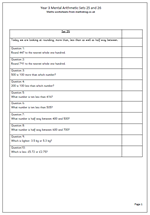Today I have published the first of our next sets of mental arithmetic for year 3. This is the first of another 12 sets for the spring term, which will build up to another 24 pages of 10 questions.

This week I am looking at rounding, using terms such as ‘more than’ and ‘less than’ as well as finding numbers half way between two other numbers.

The rounding questions are straightforward and a good check to see if children understand this idea.

Watch out for the question, ‘500 is 100 more than which number?’ Many children will not think how this has been phrased and answer 600, when the correct answer is 400.

Finding ten less than a number which is just over the hundreds boundary (eg ten less than 207) can also prove tricky. There are several approaches, including

Taking ten from the 20 tens in 207, leaving 19 tens and 7 or 197.

Counting back 7 to 200 and then another 3 to 197.